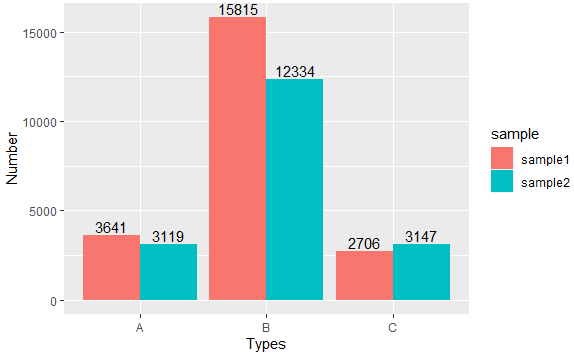3 views

I've found this, How to put labels over geom_bar in R with ggplot2, but it just put labels(numbers) over only one bar.

Here is, let's say, two bars for each x-axis, how to do the same thing?

My data and code look like this:

dat <- read.table(text = "sample Types Number

sample1 A   3641

sample2 A   3119

sample1 B   15815

sample2 B   12334

sample1 C   2706

library(ggplot2)

bar <- ggplot(data=dat, aes(x=Types, y=Number, fill=sample)) +

geom_bar(position = 'dodge') + geom_text(aes(label=Number))

Then, we'll get:It seems that the number of texts is also positioned in the "dodge" pattern. I've searched geom_text manual to find some information, but cannot make it work.

Suggestions?

+1 vote
by

To put the labels over the bars for each bar, you can use the following:

ggplot(data=dat, aes(x=Types, y=Number, fill=sample)) +

geom_bar(position = 'dodge', stat='identity') +

geom_text(aes(label=Number), position=position_dodge(width=0.9), vjust=-0.25)

Output: